# A Rapid Method for Modeling a Variable Cycle Engine

Engineering

## Your institution must subscribe to JoVE's Engineering section to access this content.

### Summary

Here, we present a protocol to build a component-level mathematical model for a variable cycle engine.

Yu, B., Miao, R., Shu, W. A Rapid Method for Modeling a Variable Cycle Engine. J. Vis. Exp. (150), e59151, doi:10.3791/59151 (2019).

### Abstract

The variable cycle engines (VCE) that combine the advantages of turbofan and turbojet engines, are widely considered to be the next generation aircraft engines. However, developing VCE requires high costs. Thus, it is essential to build a mathematical model when developing an aircraft engine, which may avoid a large number of real tests and reduce the cost dramatically. Modeling is also crucial in control law development. In this article, based on a graphical simulation environment, a rapid method for modeling a double bypass variable cycle engine using object-oriented modeling technology and modular hierarchical architecture is described. Firstly, the mathematical model of each component is built based on the thermodynamic calculation. Then, a hierarchical engine model is built via the combination of each component mathematical model and the N-R solver module. Finally, the static and dynamic simulations are carried out in the model and the simulation results prove the effectiveness of the modeling method. The VCE model built through this method has the advantages of clear structure and real-time observation.

### Introduction

Modern aircraft demands bring great challenges to the propulsion system, which need more intelligent, more efficient or even more versatile aircraft engines1. Future military propulsion systems also require both higher thrust at high speed and lower specific fuel consumption at low speed1,2,3,4. In order to meet the technical requirements of future flight missions, General Electric (GE) put forward the variable cycle engine (VCE) concept in 19555. A VCE is an aircraft engine that can perform different thermodynamic cycles by changing the geometry size or position of some components6. The Lockheed SR-71 "Blackbird" powered by a J58 turboramjet VCE has held the world record for the fastest air-breathing manned aircraft since 19767. It also proved many potential advantages of supersonic flight. In the past 50 years, GE has improved and invented several other VCEs, including a double bypass VCE8, a controlled pressure ratio engine9 and an adaptive cycle engine10. These studies involved not only the general structure and performance verification, but also the control system of the engine11. These studies have proven that the VCE can work like a high bypass ratio turbofan at subsonic flight and like a low bypass ratio turbofan, even like a turbojet at supersonic flight. Thus, the VCE can realize performance matching under different flight conditions.

When developing a VCE, a large amount of necessary verification works will be carried out. It may cost a large amount of time and outlay if all these works are performed in a physical way12. Computer simulation technology, which has already been adopted in developing a new engine, can not only reduce the cost greatly, but also avoid the potential risks13,14. Based on computer simulation technology, the development cycle of an engine will be reduced to nearly half, and the number of equipment required will be reduced dramatically15. On the other hand, simulation also plays an important role in the analysis of the engine behavior and control law development. For simulating the static design and off-design performance of engines, a program called GENENG16 was developed by the NASA Lewis Research Center in 1972. Then the research center developed DYNGEN17 derived from GENENG, and DYNGEN could simulate the transient performance of a turbojet and the turbofan engines. In 1989, NASA put forward a project, called Numerical Propulsion System Simulation (NPSS), and it encouraged researchers to construct a modular and flexible engine simulation program through the use of object-oriented programming. In 1993, John A. Reed developed the Turbofan Engine Simulation System (TESS) based on the Application Visualization System (AVS) platform through object-oriented programing18.

Meanwhile, rapid modeling based on graphical programming environment is being used gradually in simulation. The Toolbox for the Modeling and Analysis of Thermodynamic Systems (T-MATS) package developed by NASA is based on Matlab/Simulink platform. It is open source and allows users to customize built-in component libraries. T-MATS offers a friendly interface to users and it is convenient to analyze and design the built-in JT9D model19.

In this article, the dynamic model of a type of VCE has been developed here using Simulink software. The modeling object of this protocol is a double bypass VCE. Its schematic layout is shown in Figure 1. The engine can work in both single and double bypass modes. When the Mode Select Valve (MSV) is open, the engine performs better at subsonic conditions with a relatively large bypass ratio. When the Mode Select Valve is closed, the VCE has a small bypass ratio and a better supersonic mission adaptability. To further quantize performance of the engine, a double bypass VCE model is built based on component-level modeling method.

### Protocol

1. Preparation before modeling

1. Obtain design point performance.
1. Open Gasturb 13. Select Variable Cycle Engine.
2. Click on Basic Thermodynamics. Select Cycle Design. Open DemoVarCyc.CVC.
3. Obtain the engine design point performance. These are shown on the right side of the window.
2. Obtain component maps.
1. Open Gasturb 13. Select Variable Cycle Engine.
2. Click on Off Design. Select Standard Maps. Open DemoVarCyc.CVC.
3. Click on Off Design Point. Then select LPC, IPC, HPC, HPT and LPT; thus, all components maps are obtained.

2. Model each component of the VCE20,21,22

1. Model a single component of a VCE. Take the high-pressure compressor as an example.
1. Open Matlab. Click on Simulink. Double click on Blank Model.
2. Click on Library, and place function to model.
3. Double click on Function. According to the working principle of the compressor, the thermodynamic equation of the compressor is described. Then describe the equation with the MATLAB function.
4. After finishing the MATLAB function, obtain the input and output of the compressor.
5. Use Subsystem to mask the module. Then rename it with “compressor”. Thus far, a subsystem module called “compressor” is established.
2. Use the same steps to get the subsystems of all components including inlet, fan, duct, core driven fan stage(CDFS), bypass mixer, compressor, burner, high-pressure turbine, low-pressure turbine, mixer, afterburner and nozzle.
1. Combine output of each component with input of the next component.

3. Solution of the whole model

1. Construct dynamic co-working equations of whole model.
1. Construct dynamic co-working equations. Construct the following 6 independent co-working equations.
2. Determine the flow balance equation for inlet and outlet of burner: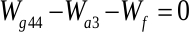Wa3: compressor outlet section air flow, Wf: burner fuel flow, Wg44: high-pressure turbine inlet gas flow.
3. Determine the flow balance equation for inlet and outlet of low-pressure turbine: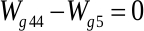Wg44: low-pressure turbine inlet section gas flow, Wg5: low-pressure turbine outlet gas flow.
4. Determine the flow balance equation for inlet and outlet of nozzle:Wg7: nozzle inlet gas flow, Wg9: nozzle outlet gas flow.
5. Determine the static pressure balance equation for inlet of rear mixer:Ps163: static pressure of main outer bypass outlet, Ps63: static pressure of inner bypass outlet.
6. Determine the flow balance equation of fan inlet and outlet:Wa2: fan inlet air flow, Wa21: CDFS inlet air flow, Wa13: sub-outer bypass inlet air flow
7. Determine the flow balance equation of CDFS outlet: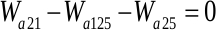Wa21: CDFS inlet air flow, Wa125: CDFS bypass inlet air flow, Wa25: compressor inlet air flow.
8. The above 6 independent equations constitute the following equations.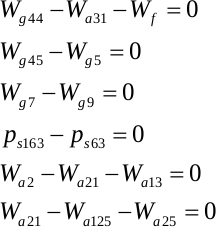2. Use the N-R iteration solver in TMATS to solve the above equations.
1. Before using the solver to solve the co-working equations, set the N-R iteration solver. According to the modeling process, select the following 6 initial guesses: component map auxiliaries line of fan, CDFS, high-pressure compressor, high-pressure turbine and low-pressure turbine β1, β2, β3, β4, β5, sub-outer bypass inlet flow.

### Representative Results

In order to prove the validity of the simulation model, several typical performance parameters selected in static and dynamic simulations are compared with the data in Gasturb.

In a static simulation, we compare several key performance parameters of the model with these parameters in Gasturb to verify the accuracy of the static model. Table 2 shows the result of the comparison at the design point with H=0 m, Ma=0, Wf=0.79334 kg/s under a double bypass operating mode. According to the comparison, the maximum error of performance parameters between the model and Gasturb is the EPR (engine pressure ratio), which is below 2%. Table 3 shows the result of the comparison at the off-design point with H=0 m, Ma=0, Wf=0.91032 kg/s under a single bypass operating mode. Under this condition, the maximum error here is the rotational speed of low-pressure shaft, which is just below 4%. The performance parameters of both models are almost the same. Thus, the two comparison results prove that the model is accurate, and the protocol is effective at the design point.

In a dynamic simulation, with the purpose of verifying the correctness of the transition state model, we simulated two typical dynamic processes including acceleration/deceleration simulation and mode switching simulation. The acceleration/deceleration simulation are processed under a double bypass mode with H=0 m, Ma=0. Figure 2a shows the input of the fuel flow. Figure 2b, Figure 2c and Figure 2d show the response of the rotational speed, air flow and temperature before the turbine, so the model is able to perform acceleration/deceleration simulation. A mode switching simulation is carried out from the double bypass mode to the single bypass mode with H=0 m, Ma=0. As shown in the Figure 3, the VCE operating mode is switched from the single bypass mode to the double bypass mode at 5 s. In order to prevent the engine from exceeding the limited speed during the switching process, a single-variable closed-loop control is applied to the rotational speed of high-pressure shaft. Figure 3b shows that the rotational speed of high-pressure shaft is nearly unchanged during switching. Similarly, Figure 3a, Figure 3b, Figure 3c and Figure 3d show the response of the fuel flow, rotational speed, air flow and temperature before the turbine. During the two-dynamic simulation, the model can run correctly.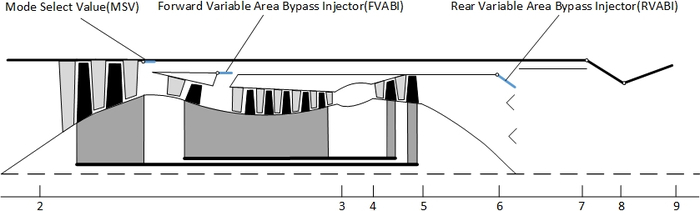Figure 1: Schematic diagram of overall structure of variable cycle engine.
A VCE contains a fan, a CDFS, a compressor, a burner, a turbine, a mixer, an afterburner and a nozzle. The fan and CDFS are driven by the low-pressure turbine. The compressor is driven by the high-pressure turbine. The numbers in Figure 1 represent the cross section of the engine. The definition of each cross sections is shown in Table 1. Please click here to view a larger version of this figure.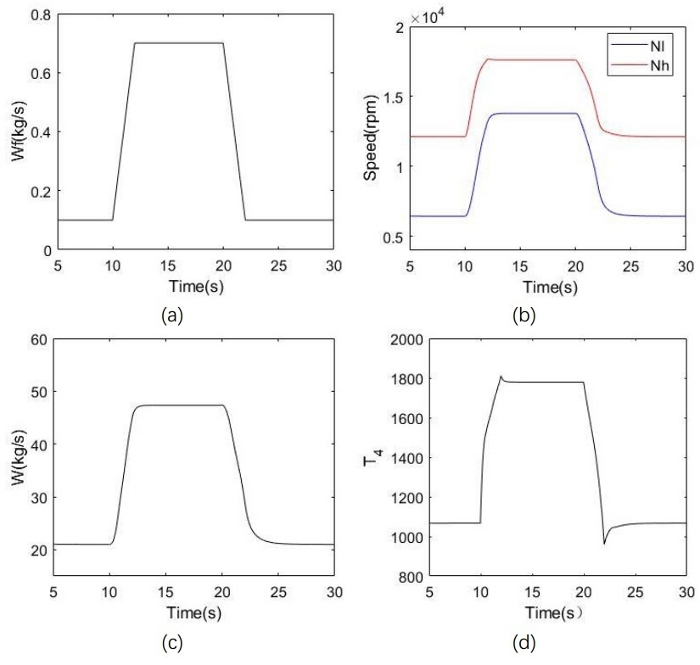Figure 2. Acceleration/deceleration simulation of VCE.
This figure presents acceleration/deceleration simulation. The fuel flow input is shown in Figure 2a. The responses of main performance parameters are shown as below. (b) The response of the high-pressure speed and the low-pressure speed. (c) The response of the air flow. (d) The response of the turbine inlet temperature. Please click here to view a larger version of this figure.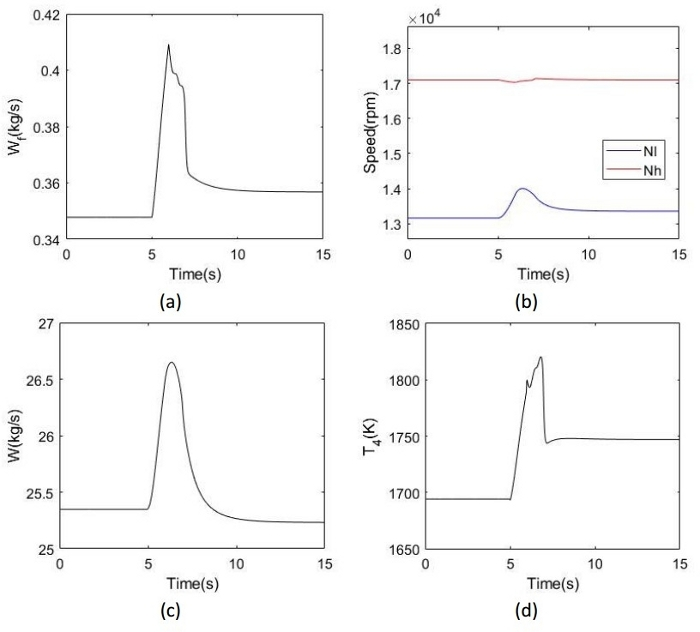Figure 3. Mode switching simulation of VCE.
This figure presents the mode switching simulation. (a) The response of the fuel flow input. (b) The response of the high-pressure speed and the low-pressure speed. (c) The response of the air flow. (d) The response of the turbine inlet temperature. Please click here to view a larger version of this figure.

 Numbe of cross section Definition 2 Fan inlet 3 Compressor outlet 4 Burner outlet 5 Low-pressure turbine outlet 6 Mixer inlet 7 Afterburner outlet 8 Nozzle thought 9 Nozzle outlet

Table 1: Definition of all cross sections. The cross-section definitions of the variable cycle engine adopted in this protocol are shown in Table 1.

 Parameter Model Gasturb 13 Error(%) Nl(RPM) 14711 14600 0.76 Nh(RPM) 18060 18000 0.33 T4(K) 1866 1850 0.86 FN(KN) 38.18 37.98 0.53 EPR 4.1653 4.2436 1.85

Table 2. Comparison of the design point of the double bypass. Several key performance parameters of model are compared with those parameters in Gasturb at the design point with H=0 m, Ma=0, Wf=0.79334 kg/s.

 Parameter Model Gasturb 13 Error(%) Nl(RPM) 15544 15033 3.4 Nh(RPM) 18123 18000 0.68 T4(K) 2036 2002 1.7 FN(KN) 41.23 40.68 1.35 EPR 4.2419 4.2894 1.11

Table 3. Comparison of the off-design point of the single bypass. Several key performance parameters are compared at the off-design point with H=0 m, Ma=0, Wf=0.91032 kg/s.

### Discussion

Based on a graphical simulation environment, a VCE component-level model can be built rapidly through modular hierarchical architecture and object-oriented modeling technology. It offers a friendly interface to users and it is convenient to analyze and design the model19.

The main limitation of this method is the execution efficiency of the model. Because the model is written in scripting language, the model needs to be recompiled every time it runs. Thus, the execution efficiency is not as good as the system language. In view of this limitation, the next key research point is how to improve the execution efficiency of the model. Another limitation is that the initial value of the N-R iteration should be considered strictly in the model, because the N-R iteration is convergent only in a small range of deviations.

A critical step in the protocol is how to obtain the component maps accurately and use the appropriate algorithm to interpolate. Whether in Gasturb or another existing engine test data, accurate component maps are helpful to build model more accurately.

In the graphical object-oriented modeling of aeroengine, whether it is the whole engine model object, the component model object or the parameter model object of each component, it is built as an independent and encapsulable module. The connection among all component modules constitute the main part of the model framework. The internal model design of each component module is for the purpose of generality, highlighting the features of easy modification and visualization of the component model. The method presented in this paper can be used not only for VCE but also for other gas turbines23.

### Disclosures

We have nothing to disclose.

### Acknowledgments

This research was funded by the Fundamental Research Funds for the Central Universities, grant number [No. NS2018017].

### Materials

 Name Company Catalog Number Comments Gasturb GasTurb GmbH Gasturb 13 MATLAB MathWorks R2017b TMATS NASA 1.2.0